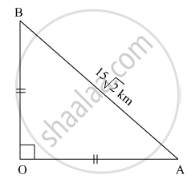Share

# Pranali and Prasad Started Walking to the East and to the North Respectively, from the Same Point and at the Same Speed. After 2 Hours Distance Between Them Was 15 √ 2 Km. Find Their Speed per Hour. - Geometry

ConceptPythagoras Theorem

#### Question

Pranali and Prasad started walking to the East and to the North respectively, from the same point and at the same speed. After 2 hours distance between them was $15\sqrt{2}$

km. Find their speed per hour.

#### SolutionIt is given that, Pranali and Prasad have same speed.
Thus, they cover same distance in 2 hours.
i.e. OA = OB

Let the speed be x km per hour.

According to Pythagoras theorem,
In ∆AOB

${AB}^2 = {AO}^2 + {OB}^2$
$\Rightarrow \left( 15\sqrt{2} \right)^2 = {AO}^2 + {OA}^2$
$\Rightarrow 450 = 2 {AO}^2$
$\Rightarrow {AO}^2 = \frac{450}{2}$
$\Rightarrow {AO}^2 = 225$
$\Rightarrow AO = 15 km$
$\Rightarrow BO = 15 km$

$\text{Speed} = \frac{Distance}{Time}$
$= \frac{15}{2}$
$= 7 . 5 \text{km per hour}$

Is there an error in this question or solution?

#### APPEARS IN

Balbharati Solution for Balbharati Class 10 Mathematics 2 Geometry (2018 to Current)
Chapter 2: Pythagoras Theorem
Problem Set 2 | Q: 10 | Page no. 45

#### Video TutorialsVIEW ALL 

Solution Pranali and Prasad Started Walking to the East and to the North Respectively, from the Same Point and at the Same Speed. After 2 Hours Distance Between Them Was 15 √ 2 Km. Find Their Speed per Hour. Concept: Pythagoras Theorem.
S